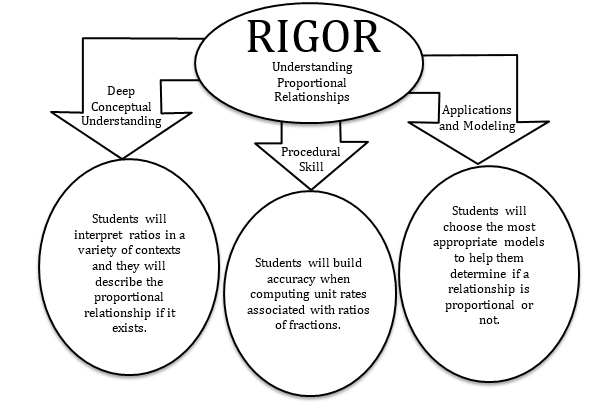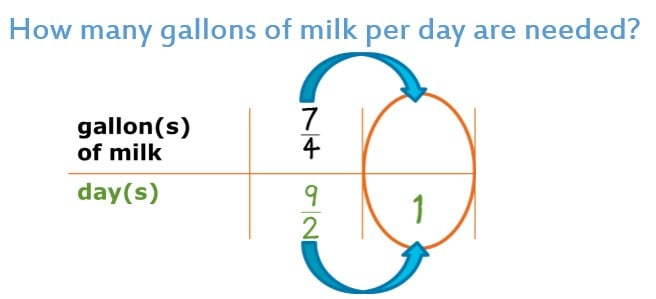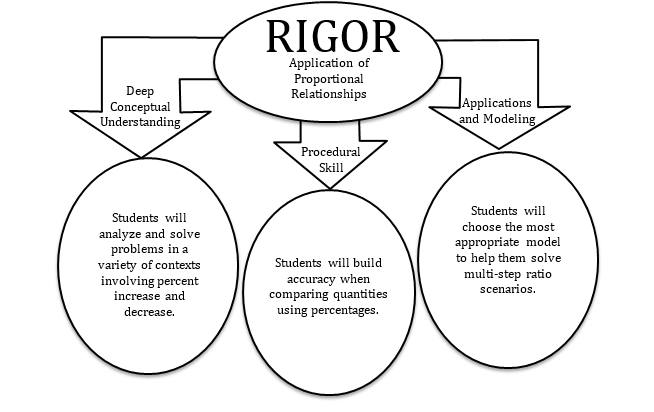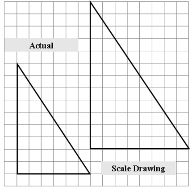# Mathematics Support

### Ratios and Proportional Relationships

Understanding Proportional Relationships

Application of Proportional Relationships

## Understanding Proportional Relationships

### Students will be able to...

• Reason about and compute unit rates associated with ratios of fractions, including ratios of lengths, areas and other quantities measured in like or different units.
• Decide whether two quantities are in a proportional relationship, e.g., by testing for equivalent ratios in a table or graphing on a coordinate plane and observing if the graph is a straight line through the origin.
• Identify the constant of proportionality (unit rate) in tables, graphs, equations, diagrams, and verbal descriptions of proportional relationships.
• Represent proportional relationships by equations.
• Explain what a point (x, y) on the graph of a proportional relationship means in terms of the situation, with special attention to the points (0, 0) and (1, r) where r is the unit rate.

*Instructional videos in the hyperlinks above are meant to support C2.0 content, but may use vocabulary or strategies not emphasized by MCPS.

The Common Core State Standards require a balance of three fundamental components that result in rigorous mathematics acquisition: deep conceptual understanding, procedural skill, and mathematical applications and modeling.### In school, your child will…

• Reason about and compute unit rates associated with ratios of fractions, including ratios of lengths, areas, and other quantities measured in like or different units.
• Decide whether two quantities are in a proportional relationship.
•  How can you determine if the number of gallons needed is proportional to the number of days?• Represent proportional relationships by equations.
• Explain what a point (x, y) on the graph of a proportional relationship means in terms of the situation.•  Explain how the graph shows or does not show the two quantities being proportional to each other.
• What does the ordered pair (0, 0) represent in the context of the problem? (300, 300)?

### At home, your child can…

• Determine the amount of ingredients needed when modifying recipes.
•  If our recipe calls for 2 1/2 cup of flour for every 3/4 cup of brown sugar, how much flour is needed if we used 1 cup of brown sugar?
•  Determine the unit price of an item at the grocery store.
•  If a 32 oz. bottle of Gatorade costs \$0.88, what is the price per ounce?
•  Decide whether two quantities are in a proportional relationship.
• the price of a movie ticket compared to the age of the person
• the number of hours worked and the amount of money earned

*Additional Practice links support C2.0 content, but may use vocabulary or strategies not emphasized by MCPS.

## Application of Proportional Relationships

### Students will be able to...

• Solve problems involving scale drawings of geometric figures.
• Identify the constant of proportionality (unit rate) in tables, graphs, equations, diagrams, and verbal descriptions of proportional relationships.
• Represent proportional relationships by equations.
• Use proportional relationships to solve multistep ratio and percent problems. Examples: simple interest, tax, markups and markdowns, gratuities and commissions, fees, percent increase and decrease, percent error.
• Understand that rewriting an expression in different forms in a context can shed light on the problem and how the quantities in it are related.

*Instructional videos in the hyperlinks above are meant to support C2.0 content, but may use vocabulary or strategies not emphasized by MCPS.

The Common Core State Standards require a balance of three fundamental components that result in rigorous mathematics acquisition: deep conceptual understanding, procedural skill, and mathematical applications and modeling.### In school, your child will…

• Solve problems involving scale drawings of geometric figures, including computing actual lengths and areas from a scale drawing and reproducing a scale drawing at a different scale.• Represent proportional relationships by equations.
• (0.12)s = 6 and 12/100 = 6/s, where s represents the total number of students.

• Use proportional relationships to solve multistep ratio and percent problems.
• Understand that rewriting an expression in different forms in context can shed light on the scenario and how the quantities in it are related.
• 15% gratuity on a \$30 meal.
• 0.15 x 30 = g, where g is the amount of the gratuity
• 30 (1 + 0.15) = t, where t is the total amount (meal and gratuity)

### At home, your child can...

• Interpret the scale on a map when planning an upcoming trip. Use the given scale to estimate the distance of your trip.
• If the trail we want to hike is 2 inches on the map, how can we use the scale to determine the length of the trail in miles?

• Determine the amount of tax that will be due when grocery shopping.
• If the subtotal of the grocery bill for the week is \$150.62, and MD State sales tax is 6%, what is the total bill?

• Determine the amount of a posted discount and calculate the new price.
• Determine the final price of an item after applying a given discount and sales tax.
• Determine the gratuity amount when eating out.